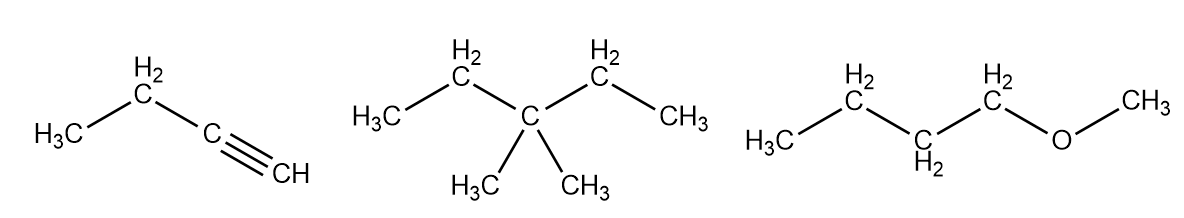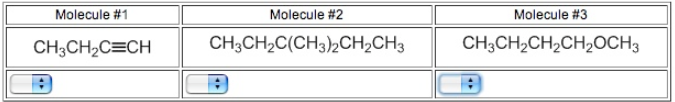# Problem: How many types of nonequivalent protons are present in each of the following molecules?

###### FREE Expert Solution

For this problem, we have to count the types of nonequivalent protons present in each molecule:• Recall that there are as many signals on each spectrum as there are unique, non-equivalent protons
• In this case, let's assume that Hs bound to the same C is equivalent
83% (151 ratings)###### Problem Details

How many types of nonequivalent protons are present in each of the following molecules?